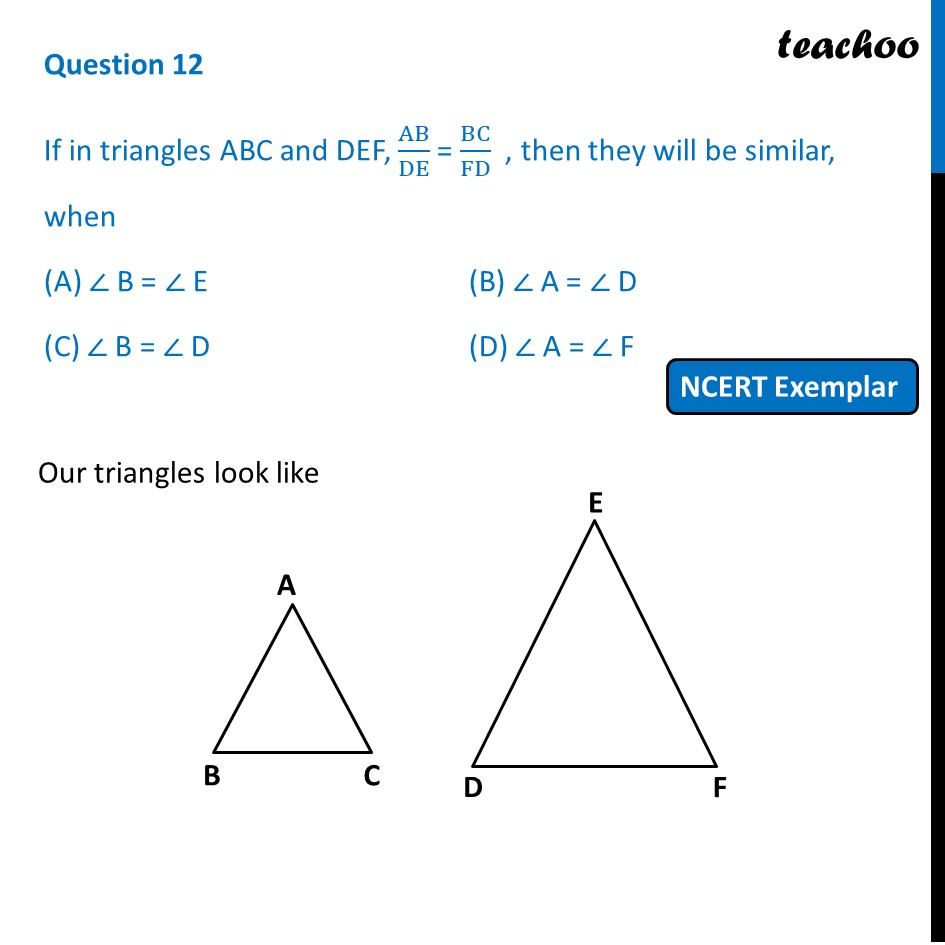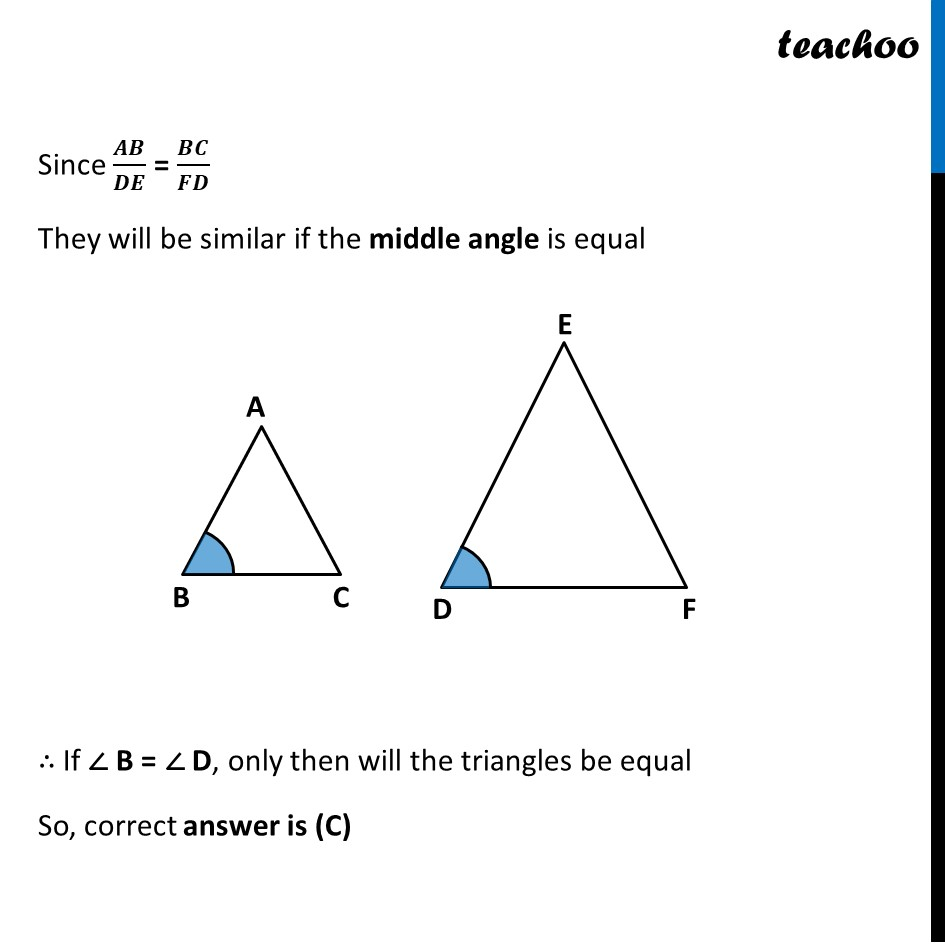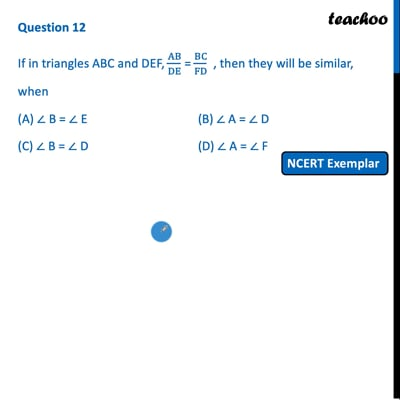NCERT Exemplar - MCQ

Chapter 6 Class 10 Triangles
Serial order wise

## (D) ∠ A = ∠ FThis video is only available for Teachoo black users

Introducing your new favourite teacher - Teachoo Black, at only ₹83 per month

### Transcript

Question 12 If in triangles ABC and DEF, AB/DE = BC/FD , then they will be similar, when (A) ∠ B = ∠ E (B) ∠ A = ∠ D (C) ∠ B = ∠ D (D) ∠ A = ∠ F Our triangles look like Since 𝑨𝑩/𝑫𝑬 = 𝑩𝑪/𝑭𝑫 They will be similar if the middle angle is equal ∴ If ∠ B = ∠ D, only then will the triangles be equal So, correct answer is (C)